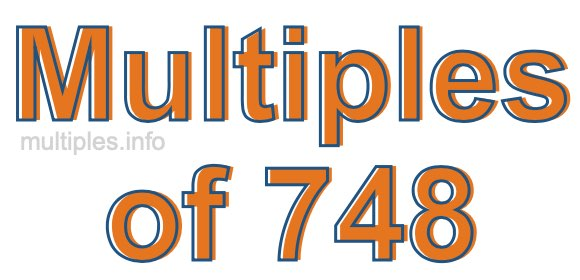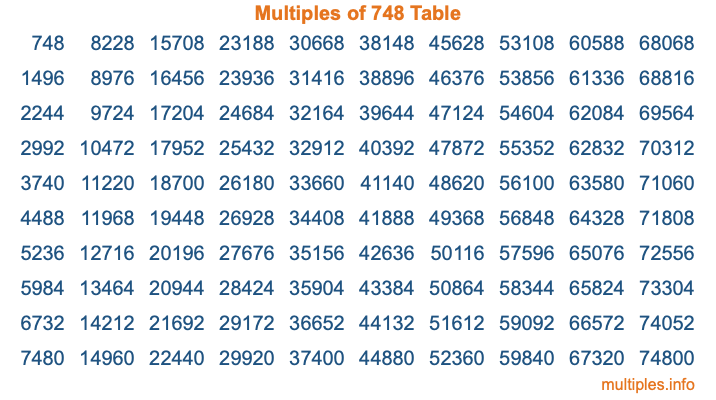Multiples of 748Welcome to the Multiples of 748 page. Here we will first teach you everything you will ever need to know about the multiples of 748, and then give you a study guide summary of everything we taught you to make sure you remember it all. Use this page to look up facts and learn information about the multiples of 748. This page will make you a multiples of seven hundred forty-eight expert!

Definition of Multiples of 748
Multiples of 748 are all the numbers that when divided by 748 equal an integer. Each of the multiples of 748 are called a multiple. A multiple of 748 is created by multiplying 748 by an integer.

Therefore, to create a list of multiples of 748, you start with 1 multiplied by 748, then 2 multiplied by 748, then 3 multiplied by 748, and so on for as long as you want. Thus, the list of the first five multiples of 748 is 748, 1496, 2244, 2992, and 3740. To see a larger list of multiples of 748, see the printable image of Multiples of 748 further down on this page. We also have a category where you can choose any nth multiple of 748.

Multiples of 748 Checker
The Multiples of 748 Checker below checks to see if any number of your choice is a multiple of 748. In other words, it checks to see if there is any number (integer) that when multiplied by 748 will equal your number. To do that, we divide your number by 748. If the the quotient is an integer, then your number is a multiple of 748.

Is  a multiple of 748?

Least Common Multiple of 748 and ...
A Least Common Multiple (LCM) is the lowest multiple that two or more numbers have in common. This is also called the smallest common multiple or lowest common multiple and is useful to know when you are adding our subtracting fractions. Enter one or more numbers below (748 is already entered) to find the LCM.

Check out our LCM Calculator if you need more details about the Least Common Multiple or if you need the LCM for different numbers for adding and subtraction fractions.

nth Multiple of 748
As we stated above, 748 is the first multiple of 748, 1496 is the second multiple of 748, 2244 is the third multiple of 748, and so on. Enter a number below to find the nth multiple of 748.

th multiple of 748

Multiples of 748 vs Factors of 748
748 is a multiple of 748 and a factor of 748, but that is where the similarities end. All postive multiples of 748 are 748 or greater than 748. All positive factors of 748 are 748 or less than 748.

Below is the beginning list of multiples of 748 and the factors of 748 so you can compare:

Multiples of 748: 748, 1496, 2244, 2992, 3740, etc.

Factors of 748: 1, 2, 4, 11, 17, 22, 34, 44, 68, 187, 374, 748

As you can see, the multiples of 748 are all the numbers that you can divide by 748 to get a whole number. The factors of 748, on the other hand, are all the whole numbers that you can multiply by another whole number to get 748.

It's also interesting to note that if a number (x) is a factor of 748, then 748 will also be a multiple of that number (x).

Multiples of 748 vs Divisors of 748
The divisors of 748 are all the integers that 748 can be divided by evenly. Below is a list of the divisors of 748.

Divisors of 748: 1, 2, 4, 11, 17, 22, 34, 44, 68, 187, 374, 748

The interesting thing to note here is that if you take any multiple of 748 and divide it by a divisor of 748, you will see that the quotient is an integer.

Multiples of 748 Table
Below is an image of the first 100 multiples of 748 in a table. The table is in chronological order, column by column. The first column has the first ten multiples of 748, the second column has the next ten multiples of 748, and so on.The Multiples of 748 Table is also referred to as the 748 Times Table or Times Table of 748. You are welcome to print out our table for your studies.

Negative Multiples of 748
Although not often discussed or needed in math, it is worth mentioning that you can make a list of negative multiples of 748 by multiplying 748 by -1, then by -2, then by -3, and so on, to get the following list of negative multiples of 748:

-748, -1496, -2244, -2992, -3740, etc.

Multiples of 748 Summary
Below is a summary of important Multiples of 748 facts that we have discussed on this page. To retain the knowledge on this page, we recommend that you read through the summary and explain to yourself or a study partner why they hold true.

There are an infinite number of multiples of 748.

A multiple of 748 divided by 748 will equal a whole number.

748 divided by a factor of 748 equals a divisor of 748.

The nth multiple of 748 is n times 748.

The largest factor of 748 is equal to the first positive multiple of 748.

748 is a multiple of every factor of 748.

748 is a multiple of 748.

A multiple of 748 divided by a divisor of 748 equals an integer.

748 divided by a divisor of 748 equals a factor of 748.

Any integer times 748 will equal a multiple of 748.

Multiples of a Number
Here you can get the multiples of another number, all with the same attention to detail as we did for multiples of 748 on this page.

Multiples of
Multiples of 749
Did you find our page about multiples of seven hundred forty-eight educational? Do you want more knowledge? Check out the multiples of the next number on our list!